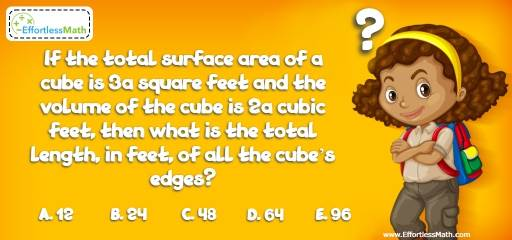# Geometry Puzzle – Challenge 75

Here you find a math puzzle to develop your child's math skills and geometry facts in a fun way.## Challenge:

If the total surface area of a cube is 3a square feet and the volume of the cube is 2a cubic feet, then what is the total length, in feet, of all the cube’s edges?

A- 12

B- 24

C- 48

D- 64

E- 96

### The Absolute Best Book to Challenge Your Smart Student!

A cube has 12 edges. Let x be the edge of the cube. So:
Surface area of the cube = 6 (one side)$$^2 = 6x^2 =3a$$
$$x^2 = \frac{1}{2} a → x = \sqrt{\frac{1}{2 }a} = (\frac{1}{2} a)^{\frac{1}{2}}$$
Volume of the cube = (one side)$$^3 = x^3 =2a → x = \sqrt{2a} = (2a)^{\frac{1}{3}}$$
Solve for a for both equations:
$$(\frac{1}{2} a)^{\frac{1}{2}} = (2a)^{\frac{1}{3}}$$
Both sides to the power of 6:
$$(\frac{1}{2} a)^3 = (2a)^2 → \frac{1}{8} a^3 = 4a^2 → \frac{1}{8} a = 4 → a = 32$$
Therefore, the volume of the cube is 64 cubic feet and one side is 4.
The total length of all the cube’s edges is 48 feet. (4 × 12 = 48)

The Best Books to Ace Algebra

### What people say about "Geometry Puzzle – Challenge 75 - Effortless Math: We Help Students Learn to LOVE Mathematics"?

No one replied yet.

X
45% OFF

Limited time only!

Save Over 45%

SAVE $40 It was$89.99 now it is \$49.99